Design of the 11011 Sequence Detector

A sequence detector accepts as input a string of bits: either 0 or 1.

Its output goes to 1 when a target sequence has been detected.

There are two basic types: overlap and non-overlap.

In an sequence detector that allows overlap, the final bits of one
sequence can be the start of another sequence.

11011 detector with overlap          X    11011011011

Z    00001001001

11011 detector with no overlap            Z    00001000001

Problem: Design a 11011 sequence detector using JK flip-flops.  Allow overlap.

Step 1 – Derive the State Diagram and State Table for the Problem

Step 1a – Determine the Number of States

We are designing a sequence detector for a 5-bit sequence, so we need 5 states.
We label these states A, B, C, D, and E.  State A is the initial state.

Step 1b – Characterize Each State by What has been Input and What is Expected

State        Has       Awaiting
A           --                11011

B           1                  1011

C           11                  011

D           110                  11

E           1101                  1

Step 1c – Do the Transitions for the Expected Sequence

Here is a partial drawing of the state diagram.  It has only the sequence
expected.  Note that the diagram returns to state C after a successful
detection; the final 11 are used again.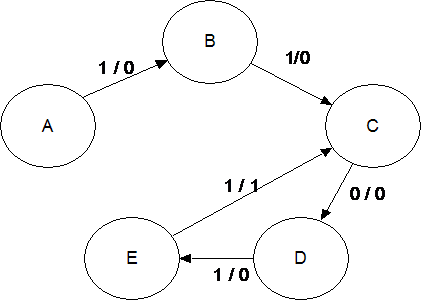Note the labeling of the transitions: X / Z.  Thus the
expected transition from A to B has an input of 1
and an output of 0.

The transition from E to C has an output of 1
denoting that the desired sequence has been detected.

The sequence is 1 1 0 1 1.

Step 1d – Insert the Inputs That Break the Sequence

The sequence is 1 1 0 1 1.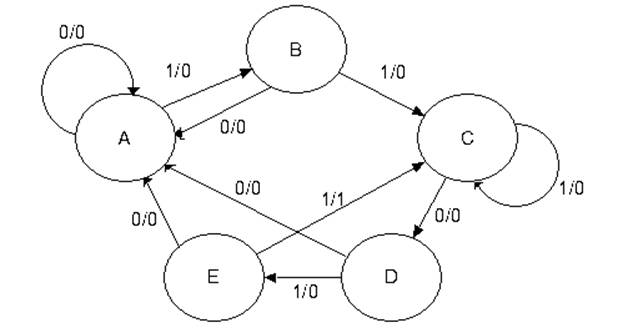Each state has two lines out of it – one line for a 1 and another line for a 0.

The notes below explain how to handle the bits that break the sequence.

State A in the 11011 Sequence Detector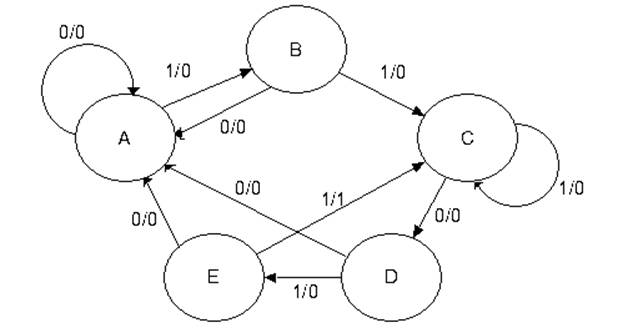A    State A is the initial state.  It is waiting on a 1.

If it gets a 0, the machine remains in state A and continues to remain
there while 0’s are input.

If it gets a 1, the machine moves to state B, but with output 0.

State B in the 11011 Sequence DetectorB    If state B gets a 0, the last two bits input were “10”.

This does not begin the sequence, so the machine goes back to state A
and waits on the next 1.

If state B gets a 1, the last two bits input were “11”.  Go to state C.

State C in the 11011 Sequence DetectorC    If state C gets a 1, the last three bits input were “111”.
It can use the last two to be the first two 1’s of the sequence 11011, so the
machine stays in state C awaiting a 0.

If state C gets a 0, the last three bits input were “110”.  Move to state D.

State D in the 11011 Sequence DetectorD    If state D gets a 0, the last four bits input were “1100”.  These 4 bits are
not part of the sequence, so we start over.

If state D gets a 1, the last four bits input were “1101”.  Go to state E.

State E in the 11011 Sequence DetectorE    If state E gets a 0, the last five bits input were “11010”.  These five bits are
not part of the sequence, so start over.

If state E gets a 1, the last five bits input were “11011”, the target sequence.
If overlap is allowed, go to state C and reuse the last two “11”.
If overlap is not allowed, go to state A, and start over.

Prefixes and Suffixes: State C

When breaking the input, we look for the largest suffix of the actual input
that is an equal–length prefix of the desired pattern.

State C, with the last input = 1.

The last three bits input were “111”.

Input    Desired Sequence.
“111”            “11011”

1 bit        “1”                  “1”           Good match        111        11011

2 bit       “11”                “11”          Good match        111        11011

3 bit      “111”              “110”         No match.           111        11011

The last two 1’s at this state can form a 2–bit prefix useable at state C.

Prefixes and Suffixes: State E

State E, with last input = 0.

The last five bits were “11010”.  No suffix of this is a prefix of the target.

Input    Desired Sequence.
“11010”          “11011”

1 bit        “0”                  “1”           No match.

2 bit       “10”                “11”          No match.

3 bit      “010”              “110”         No match.

4 bit     “1010”            “1101”        No match.

5 bit    “11010”          “11011”       No match.

Step 1e – Generate the State Table with Output

 Present State Next State / Output X = 0 X = 1 A A / 0 B / 0 B A / 0 C / 0 C D / 0 C / 0 D A / 0 E / 0 E A / 0 C / 1

Step 2 – Determine the Number of Flip-Flops Required

We have 5 states, so N = 5.  We solve the equation 2P-1 < 5 £ 2P by inspection,
noting that it is solved by P = 3.  So we need three flip-flops.

Step 3 – Assign a unique P-bit binary number (state vector) to each state.

The simplest way is to make the following assignments
A = 000
B = 001
C = 010
D = 011
E = 100

Here is a more interesting assignment.

States A and D are given even numbers.  States B, C, and E are given odd
numbers.  The assignment is as follows.

A = 000

B = 001

C = 011             States 010, 110, and 111 are not used.

D = 100

E = 101

Step 4 – Generate the Transition Table With Output

 Present State Next State / Output X = 0 X = 1 Y2Y1Y0 Y2Y1Y0 / Z Y2Y1Y0 / Z A 0  0  0 0 0 0 / 0 0 0 1 / 0 B 0  0  1 0 0 0 / 0 0 1 1 / 0 C 0  1  1 1 0 0 / 0 0 1 1 / 0 D 1  0  0 0 0 0 / 0 1 0 1 / 0 E 1  0  1 0 0 0 / 0 0 1 1 / 1

Note that bit 0 can clearly be represented by a D flip–flop with D0 = X.

Step 4a – Generate the Output Table and Equation

The output table is generated by copying from the table just completed.

 Present State X = 0 X = 1 Y2Y1Y0 0 0 0 0 0 0 0 0 0 1 0 0 0 1 1 0 0 1 0 0 0 0 1 0 1 0 1

The output equation can be obtained from inspection.
As is the case with most sequence detectors, the
output Z is 1 for only one combination of present state
and input.  Thus we get Z = X
· Y2 · Y1· Y0.

This can be simplified by noting that the state 111 does
not occur, so the answer is Z = X
· Y2 · Y0.

Step 5 – Separate the Transition Table into 3 Tables, One for Each Flip-Flop

We shall generate a present state / next state table for each of the three
flip-flops; labeled Y2, Y1, and Y0.  It is important to note that each of
the tables must include the complete present state, labeled by the
three bit vector Y2Y1Y0.

 Y2 Y1 Y0 PS Next State PS Next State PS Next State Y2Y1Y0 X = 0 X = 1 Y2Y1Y0 X = 0 X = 1 Y2Y1Y0 X = 0 X = 1 0 0 0 0 0 0 0 0 0 0 0 0 0 0 1 0 0 1 0 0 0 0 1 0 1 0 0 1 0 1 0 1 1 1 0 0 1 1 0 1 0 1 1 0 1 1 0 0 0 1 1 0 0 0 0 1 0 0 0 1 1 0 1 0 0 1 0 1 0 1 1 0 1 0 1

Match        Y1     Y2·Y0                      0         Y0                         0  1

D2 = X’·Y1 + X·Y2·Y0

D1 = X ·Y0

D0 = X

Step 6 – Decide on the type of flip-flops to be used.

The problem stipulates JK flip-flops, so we use them.

Q(T) Q(T + 1)   J       K

0          0         0       d

0          1         1       d

1          0         d       1

1          1         d       0

Steps 7 and 8 are skipped in this lecture.

Step 9 – Summarize the Equations

The purpose of this step is to place all of the equations into one location

Z = X·Y2·Y0

J2 = X’·Y1 and K2 = X’ + Y0

J1 = X·Y0 and K1 = X’

J0 = X and K0 = X’

Step 10 – Draw the Circuit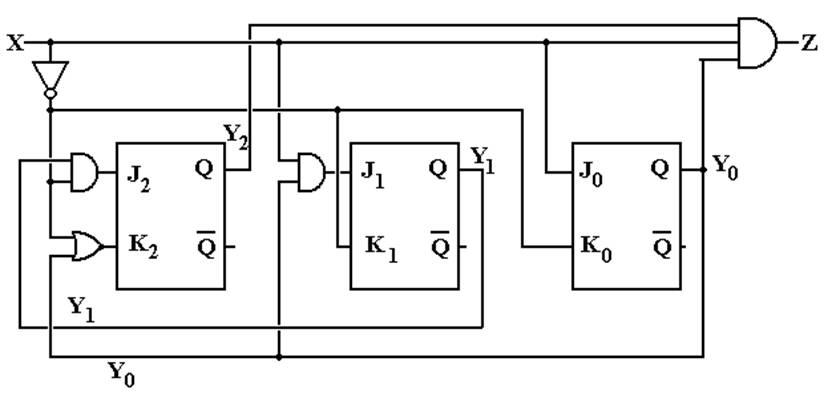Here is the same design implemented with D flip-flops.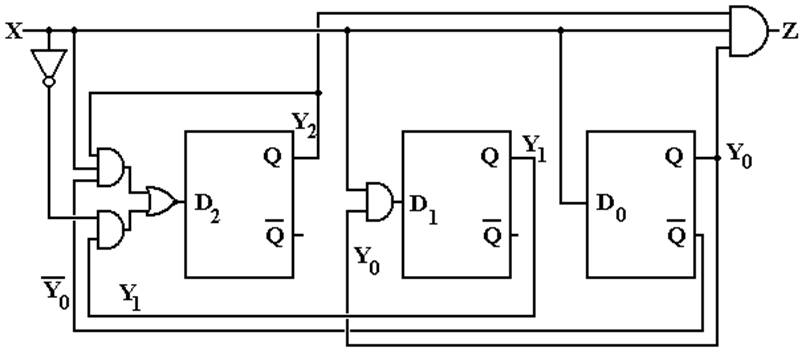More on Overlap – What it is and What it is not

At this point, we need to focus more precisely on the idea of overlap in a
sequence detector.  For an extended example here, we shall use a
1011 sequence detector.

The next figure shows a partial state diagram for the sequence detector.
The final transitions from state D are not specified; this is intentional.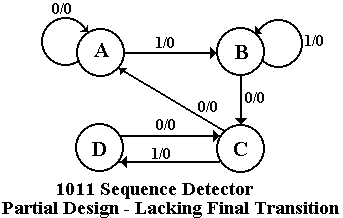Here we focus on state C and the X=0
transition coming out of state D.  By
definition of the system states,

State C – the last two bits were 10

State D – the last three bits were 101.

If the system is in state D and gets a 0 then the last four bits were 1010,
not the desired sequence. If the last four bits were 1010, the last two were
10 – go to state C.  The design must reuse as many bits as possible.

Note that this decision to go to state C when given a 0 is state D is totally
independent
of whether or not we are allowing overlap.  The question of
overlap concerns what to do when the sequence is detected, not what to
do when we have input that breaks the sequence.

Just to be complete, we give the state diagrams for the two implementations
of the sequence detector – one allowing overlap and one not allowing overlap.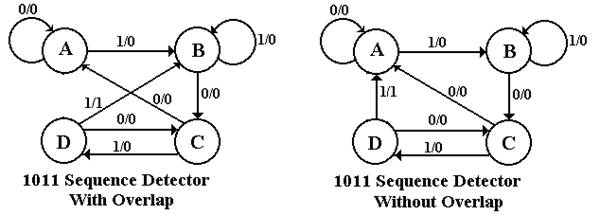The student should note that the decision on overlap does not affect designs for
handling partial results – only what to do when the final 1 in the sequence 1011 is detected.